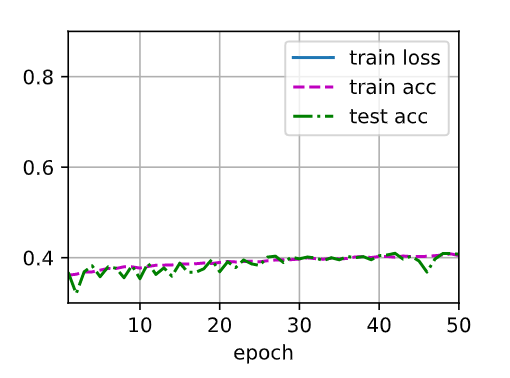# 多层感知机

1 Like

z = torch.tensor([1.0,1.5,2.], dtype=float)
b = torch.tanh(z)+1.0
c = 2.0torch.sigmoid(2.0z)
print(b.data,c.data)
c.eq(b)

tensor([1.7616, 1.9051, 1.9640], dtype=torch.float64) tensor([1.7616, 1.9051, 1.9640], dtype=torch.float64)

Out:

tensor([False, False, True])

ImportError: cannot import name ‘_check_savefig_extra_args’ from ‘matplotlib.backend_bases’ (/Library/Frameworks/Python.framework/Versions/3.8/lib/python3.8/site-packages/matplotlib/backend_bases.py)

1 Like

： ）

1 Like

!pip uninstall matplotlib

!pip install matplotlib==3.0.0

def network(x, t):
y = math.stack([x, t], axis=-1)
for i in range(8):
y = tf.layers.dense(y, 20, activation=math.tanh, name=‘layer%d’ % i, reuse=tf.AUTO_REUSE)
return tf.layers.dense(y, 1, activation=None, name=‘layer_out’, reuse=tf.AUTO_REUSE)

because there is no grad and you don’t need to set it to zero.1 Like
1 Like